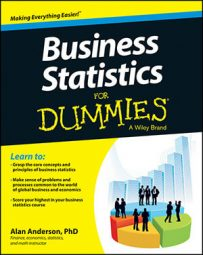An unconditional, or marginal, probability is one where the events (possible outcomes) are independent of each other. When you create a joint probability table, the unconditional probability of an event appears as a row total or a column total.

For example, say that you create a joint probability table representing the distribution of students in a business school; you classify them according to major and whether they're working on a bachelor's degree or a master's degree.

Joint Probability Table Showing the Distribution of Business Students
Degree Type Majoring in Finance Majoring in Accounting Majoring in Marketing Total
Bachelor's degree 0.26 0.36 0.18 0.80
Master's degree 0.09 0.07 0.04 0.20
Total 0.35 0.43 0.22 1.00

Based on the table, you define the following events:

• B = pursuing a bachelor's degree

• M = pursuing a master's degree

• F = majoring in finance

• A = majoring in accounting

• T = majoring in marketing

You can then find the unconditional probabilities of the following events directly from the table:

• P(B) = the probability of pursuing a bachelor's degree

• P(M) = the probability of pursuing a master's degree

• P(F) = the probability of majoring in finance

• P(A) = the probability of majoring in accounting

• P(T) = the probability of majoring in marketing

Say you want to find the probability that a randomly chosen business student is pursuing a bachelor's degree. In other words, you want to calculate P(B).

Referring to the table, you look at the first row (which refers to students pursuing their bachelor's degrees). The row total is 0.80. This is the probability that a randomly chosen student is pursuing a bachelor's degree.

Suppose you want to know the probability that a randomly chosen student is majoring in finance. In other words, you want to calculate P(F).

Referring to the table, you look at the first column (which refers to students majoring in finance). The column total is 0.35. This is the probability that a randomly chosen student is majoring in finance.

You can find the remaining unconditional probabilities in the same way. These are:

P(M) = 0.20

P(A) = 0.43

P(T) = 0.22